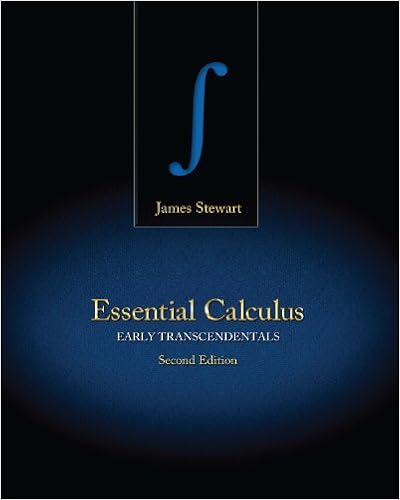# Name the intersection of pq and line k 6 name the

• Notes
• 62

This preview shows page 7 - 10 out of 62 pages.

##### We have textbook solutions for you!
The document you are viewing contains questions related to this textbook.The document you are viewing contains questions related to this textbook.
Chapter 10 / Exercise 11
Essential Calculus: Early Transcendentals
StewartExpert Verified
5. Name the intersection of ⃖ ⃗PQand line k. 6. Name the intersection of plane Aand plane B. 7.Name the intersection of line kand plane A. B k A
##### We have textbook solutions for you!
The document you are viewing contains questions related to this textbook.The document you are viewing contains questions related to this textbook.
Chapter 10 / Exercise 11
Essential Calculus: Early Transcendentals
StewartExpert Verified
E q p r 1. Understand the Problem In the diagram, you are given three lines, p , q , and r , that intersect at point B . You need to name two different planes that contain line r . 2. Make a Plan The planes should contain two points on line r and one point not on line r . 3. Solve the Problem Points D and F are on line r . Point E does not lie on line r . So, plane DEF contains line r . Another point that does not lie on line r is C . So, plane CDF contains line r . Note that you cannot form a plane through points D , B , and F . By definition, three points that do not lie on the same line form a plane. Points D , B , and F are collinear, so they do not form a plane. 4. Look Back The question asks for two different planes. You need to check whether plane DEF and plane CDF are two unique planes or the same plane named differently. Because point C does not lie on plane DEF , plane DEF and plane CDF are different planes. Monitoring Progress Help in English and Spanish at BigIdeasMath.com Use the diagram that shows a molecule of phosphorus pentachloride. G K L I J H s 8. Name two different planes that contain line s. 9. Name three different planes that contain point K. 10. Name two different planes that contain HJ .
1. WRITING Compare collinear points and coplanar points. 2. WHICH ONE DOESN’T BELONG? Which term does notbelong with the other three? 3. Name four points. 4. Name two lines. 5. Name the plane that contains points A, B, and C. 6. Name the plane that contains points A, D, and E. 7. Give two other names for ⃖ ⃗ WQ . 8. Give another name for plane V . 9. Name three points that are collinear. Then name a fourth point that is not collinear with these three points.
•••